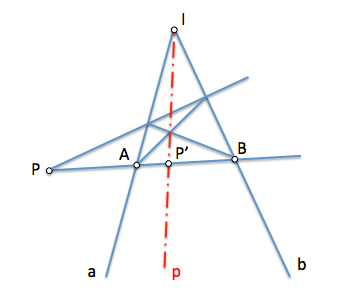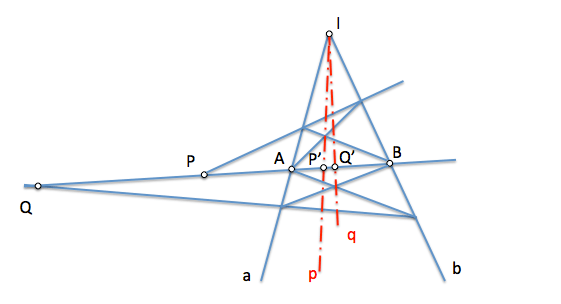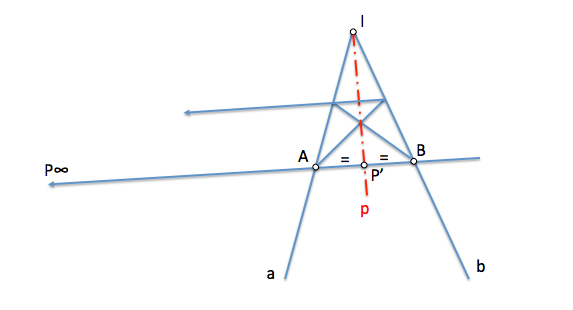# Geometría proyectiva: Conjugate directionsThe concepts of polarity we've seen when determining the Polar of a point on a line, that have allowed us to obtain the autopolar triangle of a conical to establish three different involuciuones with four points, They allow us to advance in the projective definition of its notable elements, diameters, Center and axis.

One of the basics is the of “Conjugate directions”

We can carry out previous definitions which we will be analyzing step by step based on Involutions between two superimposed series of second order and harmonious relations we have studied.

Like this, We define the following elements:

• Center of a Conic: It is the pole of the improper straight

• Conjugate polar diameters: They are polar two conjugated improper point.

• Axis a conical: They are conjugated polar diameters that are orthogonal to each other.

These definitions can be, and they are, seem very abstract and not easy to interpret. We will gradually see the concepts that allow us to understand.

### Harmonic separation and Conjugate directions

The notions of polarity are linked to the harmonic separation of elements. We say that A and B harmoniously separated to P and P’ you (ABPP ’)=-1. Let us remember that if A and B they separated harmoniously to P and P’, These also separate harmoniously to A and B, then (PP ’ AB)=-1.

The geometry of the full cuadrivertice is applicable in structures that allow us to, given three elements, determine the fourth harmonic.It change the position of point P, keeping the straight lines to and b, point P’ will also change position. Let us suppose that P passes to the position Q, the polar new will pass by Q’ determining the straight q.If we continue moving point P of the straight lines to and b, in the limit, When is P in the infinite, the harmonic conjugate P’ shall be the point of A and B. The polar p of P respecto de to and b will be your straight bisector as the Tetrad (PP ’ AB) shortlisting will become (P ’ AB) = -1.We say that the straight p addresses and address AB which contains the infinite p are conjugate directions.